# CMA Study Group

View Only#### Hossam Mohammed Atta Ahmed25 days ago#### Michael Henry23 days ago

Posted 25 days ago
Hello guys,
Can any one help me to understand and get a summary  of rules about the foh variances ?

------------------------------
Hossam Mohammed Atta Ahmed
Student
Egypt
------------------------------

• #### 2.  RE: Oveehead variance

Posted 23 days ago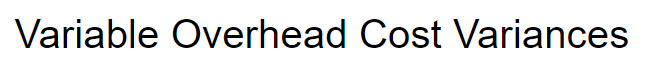The variable overhead flexible-budget variance measures the difference between actual variable overhead costs incurred and flexible-budget variable overhead amounts.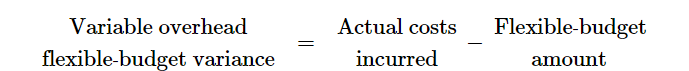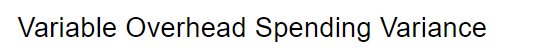The variable overhead spending variance is the difference between the actual variable overhead cost per unit of the cost-allocation base and the budgeted variable overhead cost per unit of the cost-allocation base, multiplied by the actual quantity of variable overhead cost-allocation base used.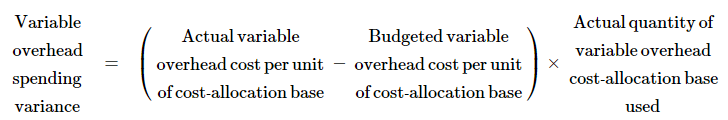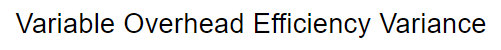The variable overhead efficiency variance is the difference between the actual quantity of the cost-allocation base used and budgeted quantity of the cost-allocation base that should have been used to produce the actual output, multiplied by the budgeted variable overhead cost per unit of the cost-allocation base.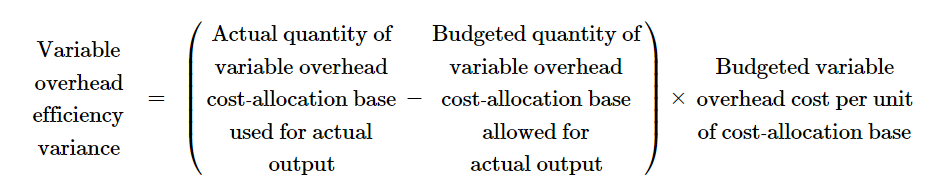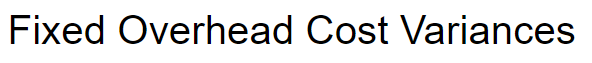The flexible-budget amount for a fixed-cost item is also the amount included in the static budget prepared at the start of the period. No adjustment is required for differences between actual output and budgeted output for fixed costs because fixed costs are unaffected by changes in the output level within the relevant range.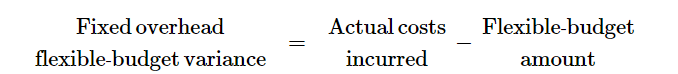There is no fixed overhead efficiency variance as the amount is fixed and does not vary with usage of a cost allocation base, therefore, the spending variance is the same as the flexible-budget variance amount.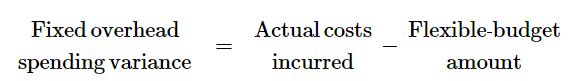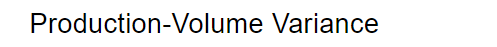The production-volume variance arises only for fixed costs. It is the difference between the budgeted fixed overhead and the fixed overhead allocated on the basis of actual output produced.  The production-volume variance, also referred to as the denominator-level variance, is the difference between the budgeted and allocated fixed overhead amounts.

Lump-sum fixed costs represent the costs of acquiring capacity. These costs do not decrease automatically if the capacity needed turns out to be less than the capacity acquired. Sometimes costs are fixed for a specific time period for contractual reasons, such as an annual lease contract for equipment. At other times, costs are fixed because capacity has to be acquired or disposed of in fixed increments, or lumps.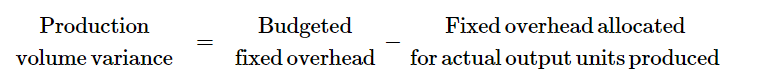SEE THE BELOW FOR AN INTEGRATED LOOK AT VARIABLE AND FIXED OVERHEAD VARIANCES (IGNORE THE NUMBERS)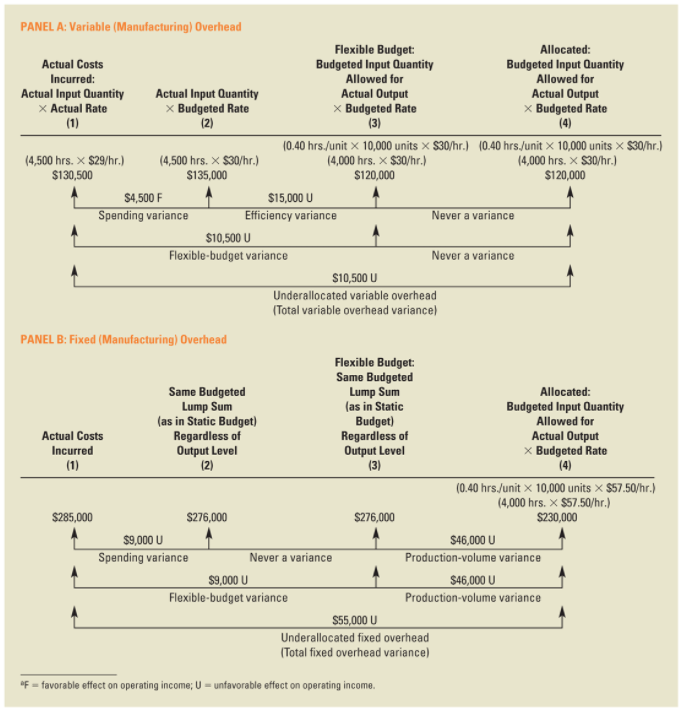------------------------------
Michael Henry
Controller
------------------------------